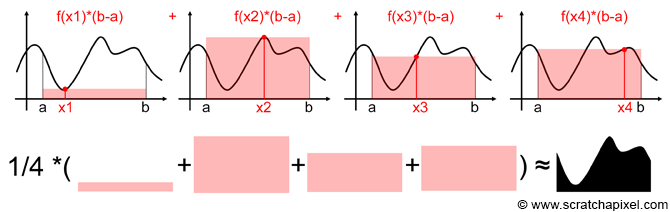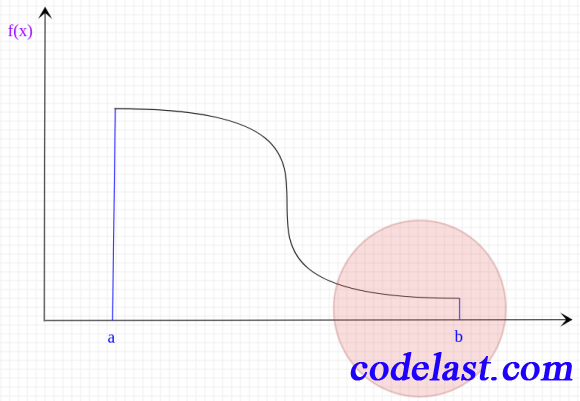## [原创] 重要性采样／Importance Sampling

• 定义

• 实例——蒙特卡罗平均值法计算定积分$\frac{1}{N}\left[ {(b - a) \times f({X_1}) + (b - a) \times f({X_2}) + \cdots + (b - a) \times f({X_N})} \right] = \frac{{b - a}}{N}\sum\limits_{i = 1}^N {f({X_i})}$

${F^N} = \frac{1}{N}\sum\limits_{i = 1}^N {\frac{{f({X_i})}}{{p({X_i})}}}$• 参考文献